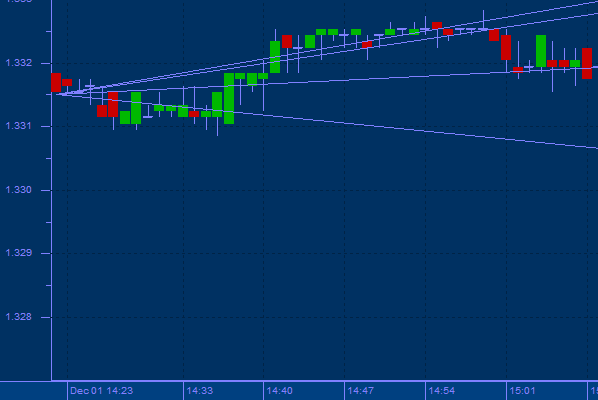top of page

## Fibonacci Fans

ibonacci numbers are a sequence of numbers in which each successive number is the sum of the two previous numbers. The Fibonacci Fan is a three-line indicator that helps determine succeeding areas of support and resistance. Those are zones in which a rise in prices may stall, or where a price decline may be stopped.

Fibonacci Fan as a line instrument is a trend .Fibonacci Fan Lines are displayed by drawing a trend line between two extreme points, for example, a trough and opposing peak. Then an “invisible” vertical line is drawn through the second extreme point. Three trend lines are then drawn from the first extreme point so they pass through the invisible vertical line at the Fibonacci levels of 38.2%, 50.0%, and 61.8%. These lines are considered to represent support and resistance levels

To create Fibonacci Fan Lines:

Identify two extreme points (highest-high and lowest-low) to be used for Fibonacci Fan Lines.

Add a Fibonacci Retracements to the chart on the two points that you select.

Usage:

As the prices pass these three fans, one can make predictions about future price movements based upon the appearance of price resistance or support at these intersection points. When the prices hold at the fan line, there is support there. If they quickly move through the fan line, do not look for support until the next fan line is met.bottom of page